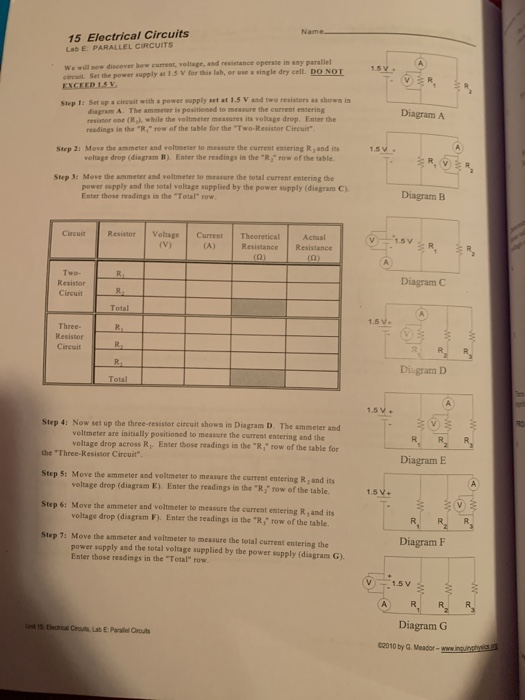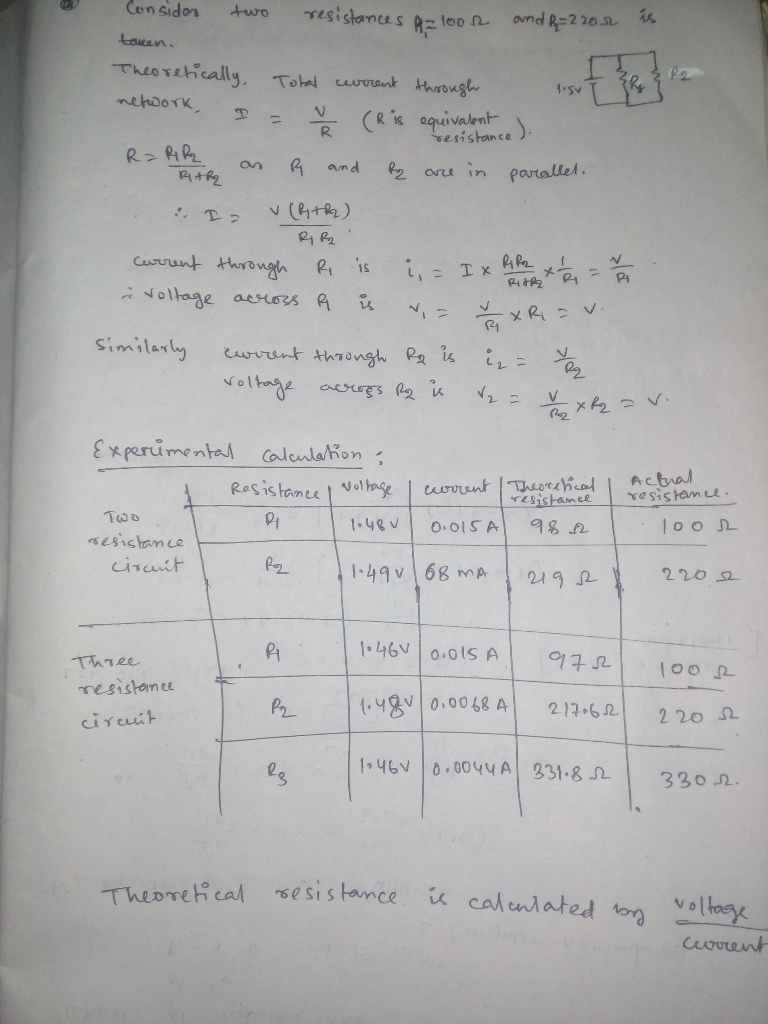Homework Help Question & Answers

15 Electrical Circuits Lab E: PARALLEL CIRCUITS Name wr w¡ฆ now discover how cument, voltage, and...15 Electrical Circuits Lab E: PARALLEL CIRCUITS Name wr w¡ฆ now discover how cument, voltage, and r cireuit. Set the power supply at 1.5 V for this lab, or use a single dry cell EXCEED ISV resistance eperate in any parallel 1.5 Do NOI Step 1: Set sp a circuit with a power supply set at 1.5 V and two resistors as shown in diagram A. The ammeser is positioned to measure the current entering Diagram A resistor one (Rj while the voltmeter messures its voltage drop. Enter the readings in the "R row of the table for the "Two-Resistor Circuit" Step 2: Move the ammeter and voltmeter to measure the current entering R and its row of the table. 1.5 veluge drop (disgram B) Enter the readings in the "R Step 3: Move the ammeter and voltmeter to mrasure the total current entering the power sspply and the total voltage supplied by the power supply (disgram C). Enter those readings in the "Total" row Diagram B Circuit Resistor Voltage Current Theoretical Two- Diagram C Resistor Circuit Total 1.5V. Three- Resistor Cireuit Di-gram D Total 1.5V+ Step 4: Now set up the three-resister circuit shows in Diagram D. The ammeter and voltmeter are initially positioned to measure the current entering and the voltage drop across R1. Enter those readings in the·R,"row of the table for the "Three-Resistor Circuit" Step S: Move the ammeter and voltmeter to measure the current entering R, and its Step 6: Move the ammeter and voltmeter to measure the current entering R, and its Step 7: Move the ammeter and voltmeter to measure the total current entering the Diagram E voltage drop (diagram E). Enter the readings in the "R row of the table. voltage drop (diagram F). Enter the readings in the "R," row of the table. power supply and the total voltage supplied by the power supply (diagram G) 1.5V+ Diagram F Enter those readings in the Total" row ˇ -:-1.5V R| R R A. Diagram G C2010 by G. Meador-wwwAdd Answer of: 15 Electrical Circuits Lab E: PARALLEL CIRCUITS Name wr w¡ฆ now discover how cument, voltage, and...
More Homework Help Questions Additional questions in this topic.

• Find the Coordinates of the missing endpoint given tha P is the midpoint of NQ, Now please helpN(2,0) P(5,2) now (2,0) (5,2) N__________P_________Q Now i am to solve for Q however, I tried to do the distance formula but I am still

Need Online Homework Help?

Get FREE EXPERT Answers
WITHIN MINUTES
Related Questions